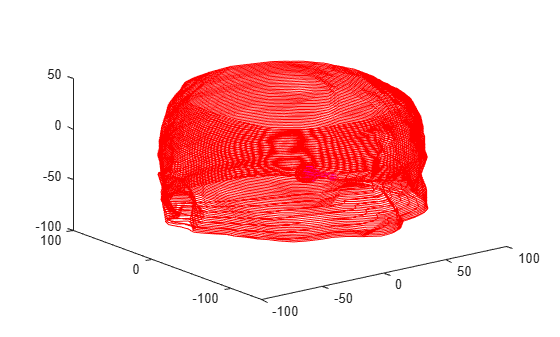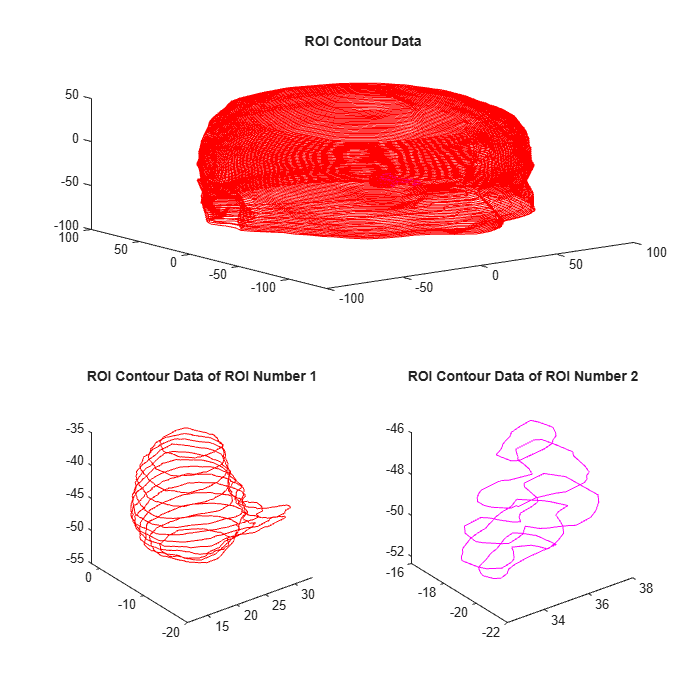# plotContour

Plot ROI contour data in DICOM-RT structure set

Since R2020a

## Syntax

``plotContour(contour)``
``plotContour(contour,number)``
``plotContour(___,ax)``
``h = plotContour(___)``

## Description

example

````plotContour(contour)` plots one or more region of interest (ROI) sequences stored in the `dicomContours` object `contour`.```

example

````plotContour(contour,number)` plots only the ROI contour data with the specified ROI number `number`.```

example

````plotContour(___,ax)` plots ROI contour data in the axes specified by `ax`, in addition to any combination of input arguments from previous syntaxes.```

example

````h = plotContour(___)` returns the graphics object handles for the plot. You can use `h` to query and modify the properties of the plot. `h` is a group object. For more information on group object properties, see Group Properties.```

## Examples

collapse all

`info = dicominfo("rtstruct.dcm");`

Extract the ROI data from the structure set and ROI contour modules of the DICOM metadata. The output is a `dicomContours` object that stores the extracted ROI data.

`contour = dicomContours(info);`

Display the `ROIs` property of the `dicomContours` object.

`contour.ROIs`
```ans=2×5 table Number Name ContourData GeometricType Color ______ _________________ ___________ _____________ ____________ 1 {'Body_Contour' } {90x1 cell} {90x1 cell} {3x1 double} 2 {'Tumor_Contour'} {21x1 cell} {21x1 cell} {3x1 double} ```

Plot all of the ROI contour data from the object.

```figure plotContour(contour)```You can also plot a specific ROI contour by using its ROI number. Plot only the ROI contour data specified by ROI number `1`.

```figure plotContour(contour,1)````info = dicominfo("rtstruct.dcm");`

Extract the ROI data from the structure set and ROI contour modules of the DICOM metadata. The output is a `dicomContours` object that stores the extracted ROI data.

`contour = dicomContours(info);`

Create a 2-by-2 tiled chart layout to display multiple plots in a figure window.

```figure("Position",[1 1 700 700]) tiledlayout(2,2)```

Create an axes object by using the `nexttile` function. The axes span across the first two columns of the tiled chart layout. Plot all of the ROI contour data on these axes.

```ax1 = nexttile(1,[1 2]); plotContour(contour,ax1) title("ROI Contour Data")```

Create a second axes object and plot only the ROI contour data specified by ROI number `1`.

```ax2 = nexttile; plotContour(contour,1,ax2) title("ROI Contour Data of ROI Number 1")```

Create a third axes object and plot only the ROI contour data specified by ROI number `2`.

```ax3 = nexttile; plotContour(contour,2,ax3) title("ROI Contour Data of ROI Number 2")````info = dicominfo("rtstruct.dcm");`

Extract the ROI data from the structure set and ROI contour modules of the DICOM metadata. The output is a `dicomContours` object that stores the extracted ROI data.

`contour = dicomContours(info);`

Plot the ROI contour data and get the parent axes. The returned parent axes is an `hggroup` object with separate handles for each ROI contour plot.

`h = plotContour(contour)`
```h = 2x1 Group array: Group (Body_Contour) Group (Tumor_Contour) ```

Add text descriptions for each ROI contour plot by using the returned handles.

```text(290,0,-400,"\leftarrow ROI number 1","Parent",h(1)) text(90,0,-500,"\leftarrow ROI number 2","FontWeight","Bold","Parent",h(2))```## Input Arguments

collapse all

ROI data, specified as a `dicomContours` object.

ROI number, specified as a numeric scalar or vector. Specifying a vector plots multiple contour sequences on the same axes.

The ROI number is specified in the `Number` column of the table returned by the `ROIs` property of the `dicomContours` object.

Data Types: `single` | `double` | `int8` | `int16` | `int32` | `int64` | `uint8` | `uint16` | `uint32` | `uint64`

Target axes, specified as a handle object returned by `axes` or `gca`.

## Output Arguments

collapse all

Graphics object handle, returned as an `hggroup` object or an array of `hggroup` objects. If you specify `number` as a vector of ROIs, `h` is an array of `hggroup` objects with each element corresponding to one ROI.

If you specify `ax`, `h` is a child of the axes `ax`. Otherwise, `h` is a child of the current axes.

## Version History

Introduced in R2020a